• 高斯混合模型聚类实战（Gaussian Mixtures）
2021-07-04 05:07:44

# 高斯混合模型聚类实战（Gaussian Mixtures）

目录

高斯混合模型聚类实战（Gaussian Mixtures）

高斯混合模型构建机器学习 数据挖掘 深度学习
更多相关内容
• 高斯混合模型聚类(Gaussian Mixture Mode，GMM)是一种概率式的聚类方法，它假定所有的数据样本x由k个混合多元高斯分布组合成的混合分布生成。 使用场景:用于平坦的结合结构，对密度估计很合适
• 简单可视化如何将EM算法用于高斯混合模型聚类。 您将需要使用matlab来运行代码。 修改datapath变量以使用另一个数据集，并修改变量K更改群集数。 特色作品： 交互式绘图，您可以在其中选择分布以生成数据
• 高斯混合模型聚类中EM算法及初始化的研究.pdf
• 基于K-means和高斯混合模型聚类的齿轮箱故障识别研究.pdf
• 这是用于聚类高斯混合建模的简单实现。 此实现旨在用于教育目的，它的实现方式使代码尽可能具有可读性，而不是尽可能高效。matlab
• ## 高斯混合模型聚类（GMM）matlab实现

万次阅读 多人点赞 2019-07-16 19:14:14
%% 高斯混合模型聚类 data = [meas(:,3), meas(:,4)]; % 手动初始条件 Mu=[0.25 1.5; 4.0 1.25; 5.5 2.0 ];%平均值设定 Sigma(:,:,1) = [1 1;1 2];%方差设定 Sigma(:,:,2) = [1 1;1 2]; Sigma(:,:,3) = [1 1;1 2];...

Gaussian Mixture Model ，就是假设数据服从 Mixture Gaussian Distribution ，换句话说，数据可以看作是从数个 Gaussian Distribution 中生成出来的。实际上，我们在 K-means 和 K-medoids 两篇文章中用到的那个例子就是由三个 Gaussian 分布从随机选取出来的。实际上，从中心极限定理可以看出，Gaussian 分布（也叫做正态 (Normal) 分布）这个假设其实是比较合理的，除此之外，Gaussian 分布在计算上也有一些很好的性质，所以，虽然我们可以用不同的分布来随意地构造 XX Mixture Model ，但是还是 GMM 最为流行。另外，Mixture Model 本身其实也是可以变得任意复杂的，通过增加 Model 的个数，我们可以任意地逼近任何连续的概率密分布。

每个 GMM 由 个 Gaussian 分布组成，每个 Gaussian 称为一个“Component”，这些 Component 线性加成在一起就组成了 GMM 的概率密度函数：
P ( x ∣ θ ) = ∑ n = 1 N c m N ( x ∣ θ m ) P(x | \theta)=\sum_{n=1}^{N} c_{m} \mathrm{N}\left(x | \theta_{m}\right)
其中Cm是权重，X是样本，θm表示第m个子模型的参数集，包含平均值以及协方差 ( μ m , Σ n ) \left(\mu_{m}, \Sigma_{n}\right)
根据上面的式子，如果我们要从 GMM 的分布中随机地取一个点的话，实际上可以分为两步：首先随机地在这 个 Component 之中选一个，每个 Component 被选中的概率实际上就是它的系数 ，选中了 Component 之后，再单独地考虑从这个 Component 的分布中选取一个点就可以了──这里已经回到了普通的 Gaussian 分布，转化为了已知的问题。

那么如何用 GMM 来做 clustering 呢？其实很简单，现在我们有了数据，假定它们是由 GMM 生成出来的，那么我们只要根据数据推出 GMM 的概率分布来就可以了，然后 GMM 的 个 Component 实际上就对应了 个 cluster 了。根据数据来推算概率密度通常被称作 density estimation ，特别地，当我们在已知（或假定）了概率密度函数的形式，而要估计其中的参数的过程被称作“参数估计”。

##### matlab代码如下：
%% 初始化工作空间
clc
clear all
close all

%% 载入数据

%% 二维数据
% 叶子长度和叶子宽度散点图(真实标记)
figure;
speciesNum = grp2idx(species);
gscatter(meas(:,3),meas(:,4),speciesNum,['r','g','b'])
xlabel('叶子长度')
ylabel('叶子宽度')
title('真实标记')
set(gca,'FontSize',12)
set(gca,'FontWeight','bold')

% 叶子长度和叶子宽度散点图（无标记）
figure;
scatter(meas(:,3),meas(:,4),150,'.')
xlabel('叶子长度')
ylabel('叶子宽度')
title('无标记')
set(gca,'FontSize',12)
set(gca,'FontWeight','bold')

%% 高斯混合模型聚类
data = [meas(:,3), meas(:,4)];

%  手动初始条件
Mu=[0.25 1.5; 4.0 1.25; 5.5 2.0 ];%平均值设定
Sigma(:,:,1) = [1 1;1 2];%方差设定
Sigma(:,:,2) = [1 1;1 2];
Sigma(:,:,3) = [1 1;1 2];
Pcom=[1/3 1/3 1/3];%混合比例
S = struct('mu',Mu,'Sigma',Sigma,'ComponentPropotion',Pcom);
GMModel = fitgmdist(data,3,'Start',S);

% 分类
T1 = cluster(GMModel,data);

% 标号调整
cen=[mean(data(T1==1,:));...
mean(data(T1==2,:));...
mean(data(T1==3,:))];
dist=sum(cen.^2,2);
[dump,sortind]=sort(dist,'ascend');
newT1=zeros(size(T1));
for i =1:3
newT1(T1==i)=find(sortind==i);
end

% 叶子长度和叶子宽度散点图(真实标记:实心圆+kmeans分类:圈)
figure;
gscatter(meas(:,3),meas(:,4),speciesNum,['r','g','b'])
hold on
gscatter(data(:,1),data(:,2),newT1,['r','g','b'],'o',10)
scatter(cen(:,1),cen(:,2),300,'m*')
hold off
xlabel('叶子长度')
ylabel('叶子宽度')
title('真实标记:实心圆+kmeans分类:圈')
set(gca,'FontSize',12)
set(gca,'FontWeight','bold')

%% 混淆矩阵 ConfusionMatrix
T2ConfMat=confusionmat(speciesNum,newT1)
error23=(speciesNum==2)&(newT1==3);
errDat23=data(error23,:)
error32=(speciesNum==3)&(newT1==2);
errDat32=data(error32,:)

%% 高斯模型等高线图
% 散点图
figure;
gscatter(meas(:,3),meas(:,4),speciesNum,['r','g','b'])
hold on
scatter(cen(:,1),cen(:,2),300,'m*')
hold off
xlabel('叶子长度')
ylabel('叶子宽度')
title('高斯模型等高线图')
set(gca,'FontSize',12)
set(gca,'FontWeight','bold')
% 叠加等高线
haxis=gca;
xlim = get(haxis,'XLim');
ylim = get(haxis,'YLim');
dinter=(max([xlim, ylim]) - min([xlim, ylim]))/100;
[Grid1, Grid2] = meshgrid(xlim(1):dinter:xlim(2), ylim(1):dinter:ylim(2));
hold on
GMMpdf=reshape(pdf(GMModel, [Grid1(:) Grid2(:)]), size(Grid1,1), size(Grid2,2));
contour(Grid1, Grid2, GMMpdf, 30);
hold off

% 混合高斯模型曲面图
figure;
surf(GMMpdf)
xlabel('叶子长度')
ylabel('叶子宽度')
title('高斯混合模型曲面图')
view(-3,65)

##### 结果显示：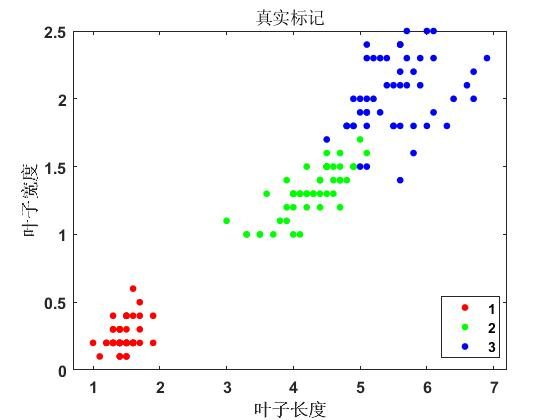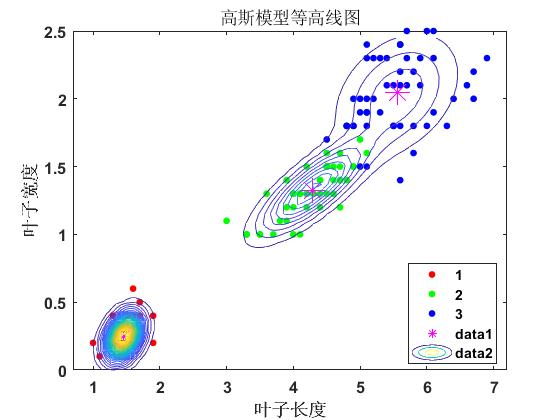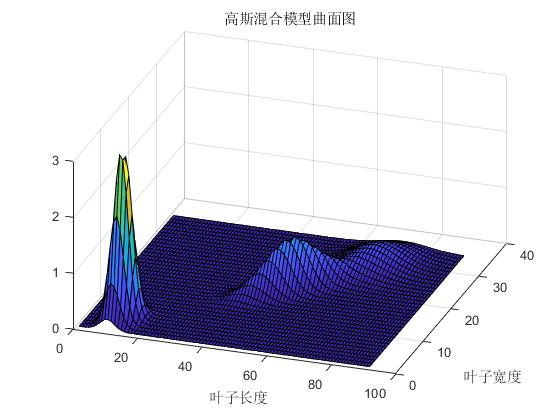展开全文• 上机器学习课学到EM算法，通过查阅西瓜书等资料理解了算法的推导过程于是形成了总结，在此进行分享。针对机器学习初学者，内容主要是：EM算法以及GMMs模型算法求解步骤的推导证明
• 基于高斯混合模型聚类的Kinect深度数据分割.pdf
• ## GMM 高斯混合模型聚类 Python实现

万次阅读 多人点赞 2018-12-26 19:46:33
大致思路就是把数据建立成k个高斯分布，EM迭代N次。最后看每个点在哪个高斯分布的概率最高，就分到那个分布。 这里的computeGamma函数，用来算第i个簇的后验概率γji，用了2层循环，效率不高，本来想向量化的，搞了...

原理也不多说了。。大致思路就是把数据建立成k个高斯分布，EM迭代N次。最后看每个点在哪个高斯分布的概率最高，就分到那个分布。

这里的computeGamma函数，用来算第i个簇的后验概率γji，用了2层循环，效率不高，本来想向量化的，搞了半天没搞出来。。干脆就先循环吧。。包括后面的fit方法也是一样。。用了很多循环。

X的shape是(n_samples,n_features)

mu的shape是(n_clusters,n_features)

sigma的shape是(n_clusters,n_features,n_features)

alpha的shape是(n_clusters,)

输出的gamma是(n_samples,n_clusters)

类方法fit里面，所有alpha初始为1/n_clusters，所有sigma协方差初始化为0.1，mu随机在样本里面选n_clusters个。

这里为了复现西瓜书的效果，所以指定mu为[[.403,.237],[.714,.346],[.532,.472]]。

import numpy as np
import matplotlib.pyplot as plt

def multiGaussian(x,mu,sigma):
return 1/((2*np.pi)*pow(np.linalg.det(sigma),0.5))*np.exp(-0.5*(x-mu).dot(np.linalg.pinv(sigma)).dot((x-mu).T))

def computeGamma(X,mu,sigma,alpha,multiGaussian):
n_samples=X.shape
n_clusters=len(alpha)
gamma=np.zeros((n_samples,n_clusters))
p=np.zeros(n_clusters)
g=np.zeros(n_clusters)
for i in range(n_samples):
for j in range(n_clusters):
p[j]=multiGaussian(X[i],mu[j],sigma[j])
g[j]=alpha[j]*p[j]
for k in range(n_clusters):
gamma[i,k]=g[k]/np.sum(g)
return gamma

class MyGMM():
def __init__(self,n_clusters,ITER=50):
self.n_clusters=n_clusters
self.ITER=ITER
self.mu=0
self.sigma=0
self.alpha=0

def fit(self,data):
n_samples=data.shape
n_features=data.shape
'''
mu=data[np.random.choice(range(n_samples),self.n_clusters)]
'''
alpha=np.ones(self.n_clusters)/self.n_clusters

mu=np.array([[.403,.237],[.714,.346],[.532,.472]])

sigma=np.full((self.n_clusters,n_features,n_features),np.diag(np.full(n_features,0.1)))
for i in range(self.ITER):
gamma=computeGamma(data,mu,sigma,alpha,multiGaussian)
alpha=np.sum(gamma,axis=0)/n_samples
for i in range(self.n_clusters):
mu[i]=np.sum(data*gamma[:,i].reshape((n_samples,1)),axis=0)/np.sum(gamma,axis=0)[i]
sigma[i]=0
for j in range(n_samples):
sigma[i]+=(data[j].reshape((1,n_features))-mu[i]).T.dot((data[j]-mu[i]).reshape((1,n_features)))*gamma[j,i]
sigma[i]=sigma[i]/np.sum(gamma,axis=0)[i]
self.mu=mu
self.sigma=sigma
self.alpha=alpha

def predict(self,data):
pred=computeGamma(data,self.mu,self.sigma,self.alpha,multiGaussian)
cluster_results=np.argmax(pred,axis=1)
return cluster_results

先用自己的GMM跑一下西瓜书的示例，结果与西瓜书上迭代50次效果一样，说明算法正确。

import pandas as pd
data=data.values

model1=MyGMM(3)
model1.fit(data)
result=model1.predict(data)
plt.scatter(data[:,0],data[:,1],c=result)
plt.scatter(model1.mu[:,0],model1.mu[:,1],marker='x',color='red')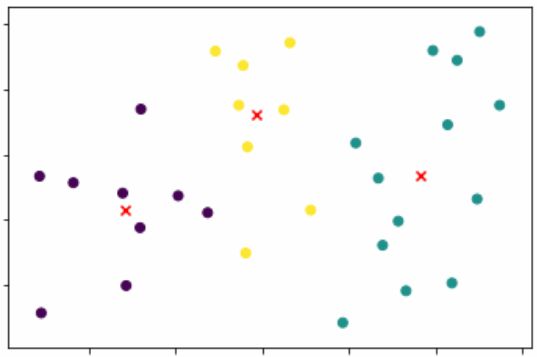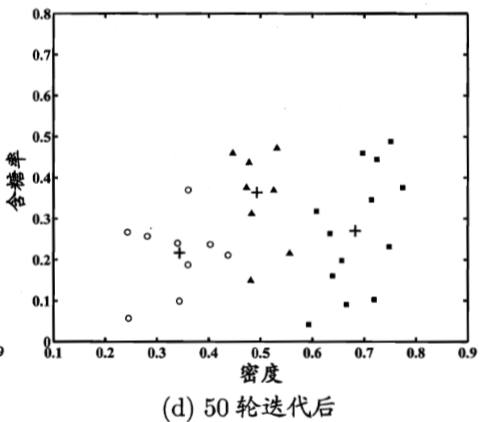然后用sklearn自带的GMM跑一下看看：由于sklearn的GMM每次初始化不一样，所以聚类的效果不一样，下图与自己实现的GMM效果一样。

from sklearn.mixture import GaussianMixture
clf=GaussianMixture(n_components=3)
clf.fit(data)
result=clf.predict(data)
plt.scatter(data[:,0],data[:,1],c=result)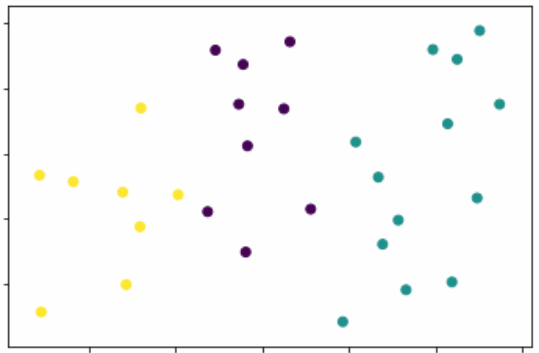最后用随机生成的5个类，来看看效果：左边是带有标签的原图，右边是聚类的图。

from sklearn.datasets import make_blobs
X, Y = make_blobs(n_samples=200, n_features=2,centers=5, cluster_std=1.0,random_state=1)
model2=MyGMM(5,100)
model2.fit(X)
result=model2.predict(X)
plt.scatter(X[:,0],X[:,1],c=Y)
plt.scatter(X[:,0],X[:,1],c=result)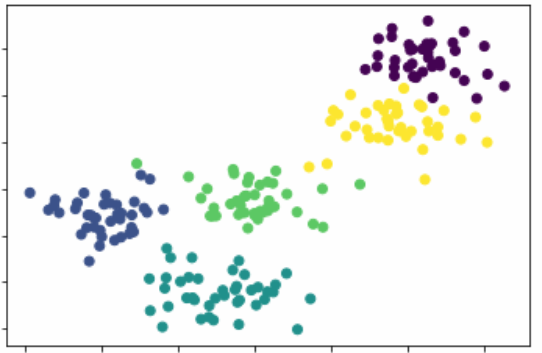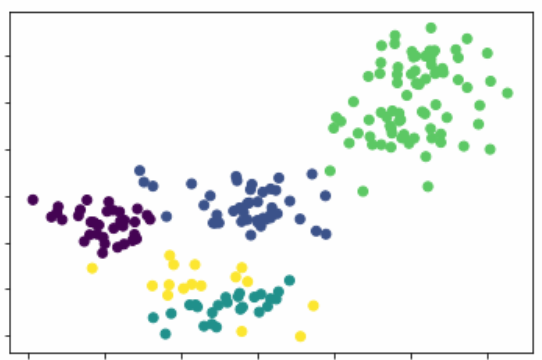右上角的数据，看起来很明显属于一个同一个高斯分布，所以GMM将其分在一起，也比较正常。倒是最下边的比较凌乱，本来要说，最下边的数据就属于同一个高斯分布，但是要聚成5个类，现在才4个类，所有就强行多出了一个，有点勉为其难了。。。

再用程序跑几次，多迭代几次，效果都差不多，右上角依旧被分在了一个类，最下边依然分不清。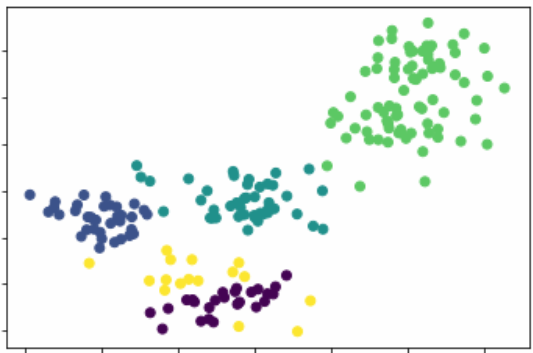改成4个类，就差不多正常了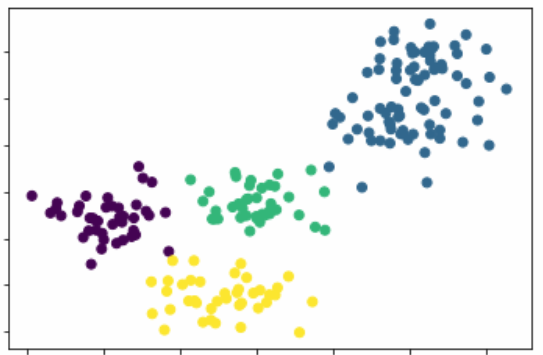展开全文GMM
• 借助图像处理技术及特征提取方法,提出了基于变换域与高斯混合模型聚类的煤岩识别方法。采用离散余弦变换和离散小波变换分别提取煤岩图像的内容和纹理信息,组成的特征向量经过高斯混合模型聚类进行分类识别。通过仿真...
• 一种快速、鲁棒的有限高斯混合模型聚类算法.pdf
• 高斯混合模型详解 聚类的方法有很多种 k-means 要数最简单的一种聚类方法了其大致思想就是把数据分为多个堆 每个堆就是一类每个堆都有一个聚类中心学习的结果就是获得这 k 个聚类中心这个中心就是这个 类中所有数据...
• 基于完整似然最短信息长度准则的高斯混合模型聚类(英文).pdf
•研究论文
• 之前写过一篇博客讲述极大似然方法， 这一方法通常适用于知道观测数据YYY，求解模型参数θ\thetaθ的...多个隐藏模型的混合，会使得普通的极大似然方法用起来不是那么方便，比如求解高斯混合模型(GMM), 隐马尔可夫...

之前写过一篇博客讲述极大似然方法， 这一方法通常适用于知道观测数据 Y Y ，求解模型参数 θ \theta 的场合，即 P ( Y ∣ θ ) P(Y|\theta)
但是，在更多场合除了模型参数是未知的外，还有隐变量 Z Z 也是未知的，即 P ( Y , Z ∣ θ ) P(Y,Z|\theta) 。多个隐藏模型的混合，会使得普通的极大似然方法用起来不是那么方便，比如求解高斯混合模型(GMM), 隐马尔可夫模型(HMM),等。这种时候，就会发觉EM算法是机器学习领域中绕不过去的一道坎。

这里，主要基于《统计学习方法》里对于高斯混合模型的讲解，实现EM算法,并给出代码和注释。
在实现模型之前，观察过其他人写的代码，包括sklearn内部的代码，相比较而言，这次的实现封装的更好，使用会更方便，结果也不错。

先描述一下实现的原理，再说代码

## 一、EM算法解高斯混合模型

假设观测数据 y 1 , y 2 , . . . , y N y_{1},y_{2},...,y_{N} 由3高斯混合模型生成，即
P ( y ∣ θ ) = ∑ k = 1 K a k ϕ ( y ∣ θ k ) P(y|\theta)=\sum_{k=1}^{K}a_{k}\phi(y|\theta_{k})
其中 θ = ( a 1 , a 2 , . . . , a k ; θ 1 , θ 2 , . . . , θ k ) \theta=(a_{1},a_{2},...,a_{k};\theta_{1},\theta_{2},...,\theta_{k}) , 我们用EM算法估计高斯混合模型的参数 θ \theta

### 1.1 EM算法的E步：确定Q函数

混合高斯模型的Q函数为：
Q ( θ , θ i ) = ∑ k = 1 K { ∑ j = 1 N ( E γ j k ) l o g a k + ∑ j = 1 N ( E γ j k ) [ l o g ( 1 / 2 π ) − l o g ( σ k ) − ( 1 / 2 σ k 2 ) ( y j − u k ) 2 ] Q(\theta,\theta^{i})=\sum_{k=1}^{K}\{\sum_{j=1}^{N}(E_{\gamma_{jk}})loga_{k}+\sum_{j=1}^{N}(E_{\gamma_{jk}})[log(1/\sqrt{2\pi})-log(\sigma_{k})-(1/2\sigma_{k}^{2})(y_{j}-u_{k})^{2}]
这里需要计算 E ( γ j k ∣ y , θ ) E(\gamma_{jk}|y,\theta) ,记为 γ ^ j k \hat{\gamma}_{jk}
γ ^ j k = a k ϕ ( y j ∣ θ k ) ∑ k = 1 K a k ϕ ( y j ∣ θ k ) , j = 1 , 2 , . . . , N ; k = 1 , 2 , . . . , K \hat{\gamma}_{jk}=\frac{a_{k}\phi(y_{j}|\theta_{k})}{\sum_{k=1}^{K}a_{k}\phi(y_{j}|\theta_{k})},j=1,2,...,N;k=1,2,...,K γ ^ j k \hat{\gamma}_{jk} 是当前模型参数下第 j j 个观测数据来自第 k k 个分模型的概率，称为分模型 k k 对观测数据 y j y_{j} 的影响度

### 1.2EM算法的M步：更新模型参数

M步极大化Q函数，更新模型参数如下：
u ^ k = ∑ j = 1 N γ ^ j k y j ∑ j = 1 N γ ^ j k , k = 1 , 2... , K \hat{u}_{k}=\frac{\sum_{j=1}^{N}\hat{\gamma}_{jk}y_{j}}{\sum_{j=1}^{N}\hat{\gamma}_{jk}},k=1,2...,K σ ^ k 2 = ∑ j = 1 N γ ^ j k ( y j − u k ) 2 ∑ j = 1 N γ ^ j k , k = 1 , 2... , K \hat{\sigma}_{k}^{2}=\frac{\sum_{j=1}^{N}\hat{\gamma}_{jk}(y_{j}-u_{k})^{2}}{\sum_{j=1}^{N}\hat{\gamma}_{jk}},k=1,2...,K a ^ k = ∑ j = 1 N γ ^ j k N , k = 1 , 2... , K \hat{a}_{k}=\frac{\sum_{j=1}^{N}\hat{\gamma}_{jk}}{N},k=1,2...,K
重复E步和M步直到模型收敛

## 二、EM算法求解高斯混合模型

from sklearn.datasets import load_digits, load_iris
from scipy.stats import multivariate_normal
from sklearn import preprocessing
from sklearn.metrics import accuracy_score
import numpy as np

class GMM_EM():
def __init__(self,n_components,max_iter = 1000,error = 1e-6):
self.n_components = n_components  #混合模型由几个gauss模型组成
self.max_iter = max_iter  #最大迭代次数
self.error = error  #收敛误差
self.samples = 0
self.features = 0
self.alpha = []   #存储模型权重
self.mu = []   #存储均值
self.sigma = []   #存储标准差

def _init(self,data):   #初始化参数
np.random.seed(7)
self.mu = np.array(np.random.rand(self.n_components, self.features))
self.sigma = np.array([np.eye(self.features)/self.features] * self.n_components)
self.alpha = np.array([1.0 / self.n_components] * self.n_components)
print(self.alpha.shape,self.mu.shape,self.sigma.shape)

def gauss(self, Y, mu, sigma):  #根据模型参数和数据输出概率向量
return multivariate_normal(mean=mu,cov=sigma+1e-7*np.eye(self.features)).pdf(Y)

def preprocess(self,data):   # 数据预处理
self.samples = data.shape
self.features = data.shape
pre = preprocessing.MinMaxScaler()
return pre.fit_transform(data)

def fit_predict(self,data):   #拟合数据
data = self.preprocess(data)
self._init(data)

weighted_probs = np.zeros((self.samples,self.n_components))
for i in range(self.max_iter):
prev_weighted_probs = weighted_probs

weighted_probs = self._e_step(data)
change = np.linalg.norm(weighted_probs - prev_weighted_probs)
if change < self.error:
break

self._m_step(data,weighted_probs)

return weighted_probs.argmax(axis = 1)

def _e_step(self,data):   # E步
probs = np.zeros((self.samples,self.n_components))
for i in range(self.n_components):
probs[:,i] = self.gauss(data, self.mu[i,:], self.sigma[i,:,:])

weighted_probs = np.zeros(probs.shape)
for i in range(self.n_components):
weighted_probs[:,i] = self.alpha[i]*probs[:,i]

for i in range(self.samples):
weighted_probs[i,:] /= np.sum(weighted_probs[i,:])

return weighted_probs

def _m_step(self,data,weighted_probs):  #M步
for i in range(self.n_components):
sum_probs_i = np.sum(weighted_probs[:,i])

self.mu[i,:] = np.sum(np.multiply(data, np.mat(weighted_probs[:, i]).T), axis=0) / sum_probs_i
self.sigma[i,:,:] = (data - self.mu[i,:]).T * np.multiply((data - self.mu[i,:]), np.mat(weighted_probs[:, i]).T) / sum_probs_i
self.alpha[i] = sum_probs_i/data.shape

def predict_prob(self,data):  #预测概率矩阵
return self._e_step(data)

def predict(self,data):  #输出类别
return self._e_step(data).argmax(axis = 1)

data = dataset['data']
label = dataset['target']

gmm = GMM_EM(3)
pre_label = gmm.fit_predict(data)
print(accuracy_score(pre_label,label))
# EM算法是对初始值敏感的，修改初始化的值会发现模型性能变化很大


## 三、资源下载

微信搜索“老和山算法指南”获取更多资源下载链接与技术交流群有问题可以私信博主，点赞关注的一般都会回复，一起努力，谢谢支持。

展开全文EM算法 python 数据集 代码实现
• 又是作业要完成高斯混合聚类，还要给出得到的混合模型参数（包括比例matlab 机器学习
• 高斯混合模型聚类算法 概念：混合高斯模型就是指对样本的概率密度分布进行估计，而估计的模型是几个高斯模型加权之和（具体是几个要在模型训练前建立好）。每个高斯模型就代表了一个类（一个Cluster）。对样本中的...算法 数据
• 用em算法估计高斯混合模型的参数，实现对N维数据的聚类
• 对西瓜书高斯混合聚类算法的实现，最终实现效果与西瓜书所展示的效果一致(ps:对混合模型的初始化完全按照西瓜书的来，读者可以稍加修改)
• 高斯分布模型GSM 多维变量X服从高斯分布时，它的概率密度函数PDF为： x是维度为d的列向量，u是模型期望，Σ是模型方差。在实际应用中u通常用样本均值来代替，Σ通常用样本方差来代替。很容易判断一个样x本...生成模型 GMM
• gmm的matlab代码高斯混合模型_聚类 高斯混合模型聚类Matlab代码 您可以选择初始化和规范化的方法。 性能指标包括ACC，ARI和ANMI。 GMM算法： 虹膜的例子 运行demo_data.m 虹膜的结果是： 迭代1，迭代次数：38，...
• 一种基于聚类高斯混合模型算法，马瑞云，，本文介绍了一种高斯混合模型算法的改进方法---将聚类算法与传统高斯混合模型结合起来的建模方法, 并同时提出的运用距离加权的矢量�说话人识别
• 简单实用的高斯混合聚类算法，适合初学者，方便好用！
• 高斯混合模型 高斯混合模型（Gaussian Mixture Model), 简称为GMM，是一个基于概率密度的模型。在这种模型中，数据点是由K个正态分布所生成的，每个正态分布都拥有自己的均值和协方差矩阵，而来自每个高斯分布的...spark java
• 本文介绍了期望最大化（Expectation-Maximization，EM）算法的公式推导，K-Means聚类算法的缺陷及改进策略，K-Means与EM算法的关系，高斯混合模型（Gaussian Mixed Model，GMM）聚类算法的公式推导。机器学习 算法 期望最大化算法
•   由于最近要做聚类算法方面的内容，看了很多资料，在高斯混合模型(GMM)这里一直没有一个让我完全推导清楚的、理解的文章。经过三天打鱼两天晒网 不懈努力，总算是有一点自己的理解，我希望尽量通俗地把GMM讲明白...机器学习 算法 人工智能...